## Sturm Function

Given a function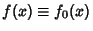, write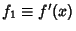and define the Sturm functions by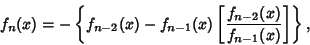(1)

where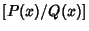is a polynomial quotient. Then construct the following chain of Sturm functions,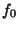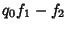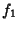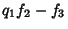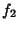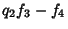(2)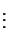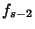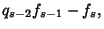known as a Sturm Chain. The chain is terminated when a constant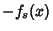is obtained.

Sturm functions provide a convenient way for finding the number of real roots of an algebraic equation with real coefficients over a given interval. Specifically, the difference in the number of sign changes between the Sturm functions evaluated at two points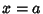and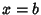gives the number of real roots in the interval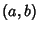. This powerful result is known as the Sturm Theorem.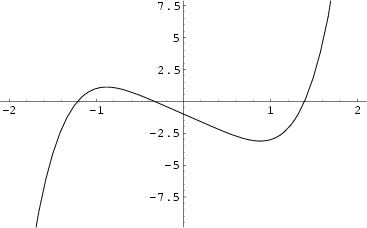As a specific application of Sturm functions toward finding Polynomial Roots, consider the function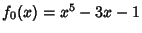, plotted above, which has roots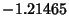,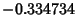,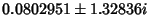, and 1.38879 (three of which are real). The Derivative is given by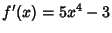, and the Sturm Chain is then given by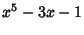(3)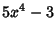(4)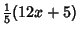(5)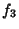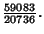(6)

The following table shows the signs of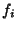and the number of sign changes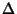obtained for points separated by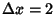.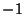11 3 01 1 1 2 1 1 1 1 0

This shows that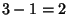real roots lie in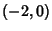, and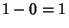real root lies in. Reducing the spacing to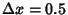gives the following table.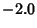11 3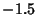11 3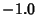1 11 2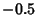11 2 0.01 1 1 0.51 1 1 1.01 1 1 1 1.5 1 1 1 1 0 2.0 1 1 1 1 0

This table isolates the three real roots and shows that they lie in the intervals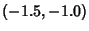,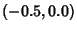, and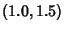. If desired, the intervals in which the roots fall could be further reduced.

The Sturm functions satisfy the following conditions:

1. Two neighboring functions do not vanish simultaneously at any point in the interval.

2. At a null point of a Sturm function, its two neighboring functions are of different signs.

3. Within a sufficiently small Area surrounding a zero point of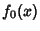,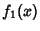is everywhere greater than zero or everywhere smaller than zero.

References

Acton, F. S. Numerical Methods That Work, 2nd printing. Washington, DC: Math. Assoc. Amer., p. 334, 1990.

Dörrie, H. Sturm's Problem of the Number of Roots.'' §24 in 100 Great Problems of Elementary Mathematics: Their History and Solutions. New York: Dover, pp. 112-116, 1965.

Press, W. H.; Flannery, B. P.; Teukolsky, S. A.; and Vetterling, W. T. Numerical Recipes in FORTRAN: The Art of Scientific Computing, 2nd ed. Cambridge, England: Cambridge University Press, p. 469, 1992.

Rusin, D. Known Math.'' http://www.math.niu.edu./~rusin/known-math/polynomials/sturm.

Sturm, C. Mémoire sur la résolution des équations numériques.'' Bull. des sciences de Férussac 11, 1929.